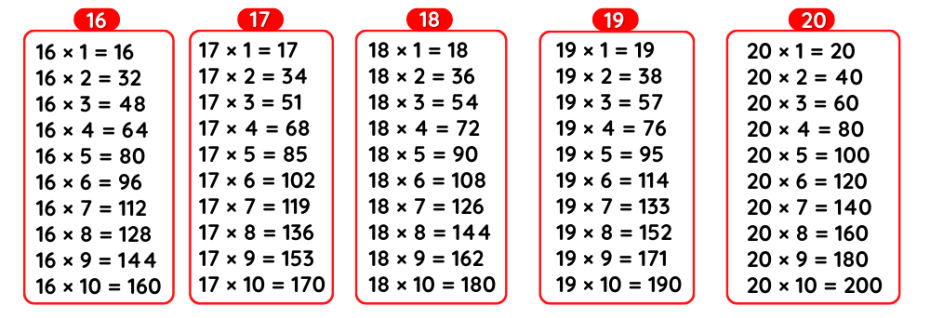# Tables 16 to 20, 16 se 20 tak table, Maths 16 to 20 tables Multiplication Chart

## Tables 16 to 20

Maths Multiplication Tables 16 to 20, Tables 16 to 20, 16 se 20 tak Table, 16 se lekar 20 tak Table, 16 to 20 Table Chart

### Table 16 to 20

 Table of 16 Table of 17 Table of 18 Table of 19 Table of 20 16 ×‌ 1 = 16 17 ×‌ 1 = 17 18 ×‌ 1 = 18 19 ×‌ 1 = 19 20 ×‌ 1 = 20 16 ×‌ 2 = 32 17 ×‌ 2 = 34 18 ×‌ 2 = 36 19 ×‌ 2 = 38 20 ×‌ 2 = 40 16 ×‌ 3 = 48 17 ×‌ 3 = 51 18 ×‌ 3 = 54 19 ×‌ 3 = 57 20 ×‌ 3 = 60 16 ×‌ 4 = 64 17 ×‌ 4 = 68 18 ×‌ 4 = 72 19 ×‌ 4 = 76 20 ×‌ 4 = 80 16 ×‌ 5 = 80 17 ×‌ 5 = 85 18 ×‌ 5 = 90 19 ×‌ 5 = 95 20 ×‌ 5 = 100 16 ×‌ 6 = 96 17 ×‌ 6 = 102 18 ×‌ 6 = 108 19 ×‌ 6 = 114 20 ×‌ 6 = 120 16 ×‌ 7 = 112 17 ×‌ 7 = 119 18 ×‌ 7 = 126 19 ×‌ 7 = 133 20 ×‌ 7 = 140 16 ×‌ 8 = 128 17 ×‌ 8 = 136 18 ×‌ 8 = 144 19 ×‌ 8 = 152 20 ×‌ 8 = 160 16 ×‌ 9 = 144 17 ×‌ 9 = 153 18 ×‌ 9 = 162 19 ×‌ 9 = 171 20 ×‌ 9 = 180 16 ×‌ 10 = 160 17 ×‌ 10 = 170 18 ×‌ 10 = 180 19 ×‌ 10 = 190 20 ×‌ 10 = 200

### 16 se 20 tak Table, 16 se lekar 20 tak Table### Multiplication Tables 16 to 20### 16 to 20 Table chart

#### Tables 11 to 20